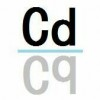【高考】推导进行n次PCR循环后目的基因的个数

2018-02-25 21:36:38

（大概也只有我这种菜鸡有闲心探究这种东西了233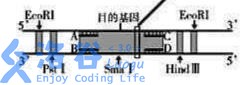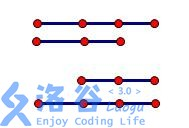(我们令上面的链是从左往右扩增的，下面反之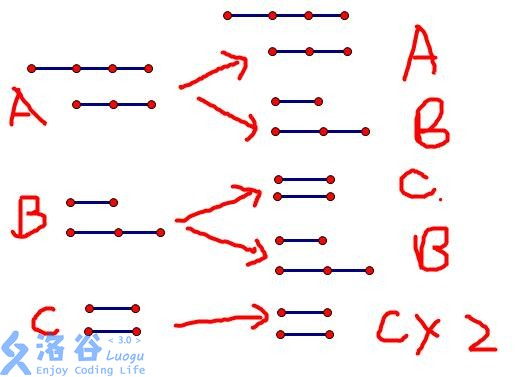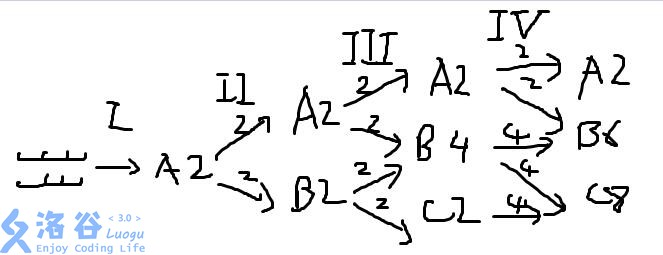（不要吐槽我鼠绘以及三张图三种画风=。=

$A_n=2 \quad B_n=A_{n-1}+B_{n-1} \quad C_n=B_{n-1}+2C_{n-1}$

$B_n=2+B_{n-1}=2(n-1)$
//$C_n=2(n-1)+2C_{n-1}=2n-2+2C_{n-1}$注意这里$B_{n-1}\neq2(n-1)$！！！
$C_n=2(n-2)+2C_{n-1}=2n-4+2C_{n-1}$

$C_n+an+b=2(C_{n-1}+a(n-1)+b)$

$C_n+2n=2(C_{n-1}+2(n-1))$

A=2，显然第n次复制后无用分子数有2n个

（这个公式大概是江苏或者浙江这种地方的考点。。。
（然后老师就把这道题删掉不做了
（是不是可以出道OI题23333
（某个外星人的DNA有n条，珂学家获取了它的一个DNA分子片段并想研究其中一段基因，现在他们想问你经过m次PCR复制之后目的基因有多少条

• star
首页